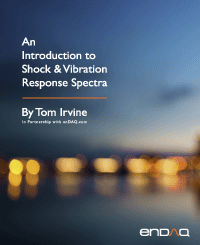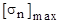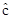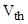Pseudo Velocity & the Stress-Velocity Relationship
By Tom Irvine
SECTION 1

# Pseudo Velocity

The Shock Response Spectrum (SRS) models the peak response of a single-degree-of-freedom (SDOF) system to a base acceleration, where the system's natural frequency is an independent variable. The absolute acceleration and the relative displacement of the SDOF system can be readily calculated. The relative velocity can also be calculated, but the common practice is to approximate the relative velocity in terms of the "pseudo velocity."

The preferred method for calculating the peak pseudo velocity is to multiply the peak relative displacement by the angular natural frequency as shown in equation (18.10). An alternate method is to divide the peak acceleration by this frequency.

SECTION 2

## Stress-Velocity RelationshipHoward A. Gaberson (1931-2013) championed the idea that dynamic stress correlated better with pseudo velocity than with either acceleration or relative displacement. Gaberson was a shock and vibration specialist who was with the U.S. Navy Civil Engineering Laboratory and later the Facilities Engineering Service Center from 1968 to 2000, mostly conducting dynamics research. He specialized in shock and vibration signal analysis and published more than 100 papers and articles. Gaberson pointed out that the kinetic energy in a mechanical system is proportional to velocity squared. The pseudo velocity is thus a measure of the stored peak energy in the system at a particular frequency and has a direct relationship to the survival or failure of the system. The following equations are taken from Reference :

Consider the stress-velocity relationship for a traveling wave in an infinite rod.

The stress s is proportional to the velocity as

The velocity depends on natural frequency, but the stress-velocity relationship does not. The lack of a frequency term in equation (1.1) was another reason that Gaberson promoted the stress-velocity relationship.

The principle can be extended to a finite rod with common boundary conditions where the peak modal stressis

The index n represents the mode number. The peak stress is mainly due to the fundamental mode in most cases. But higher modes can contribute significant stress in certain cases, such as pyrotechnic shock response.

SECTION 3
Beam Stress

Consider the bending vibration of a simply-supported beam. The modal stress due to bending is proportional to the modal velocity as follows.

The distance from the beam’s neutral axis to the outer fiber is.

Note this equation applies to other boundary condition cases per Reference .

Equation (1.3) can be simplified as follows:

Values for theconstant for typical cross-sections are:

 Cross-sectionSolid Circular 2 RectangularThe method can also be used for complex structures, but with some uncertainty. The stress-velocity formula is

The constant of proportionality K depends on geometry, etc. Bateman  claimed the following range for complex equipment 4 < K < 8. Gaberson  gave a larger range with 1 < K < 10.

Equation (19.5) could thus be used to “bound the problem” by assuming a high and low K value.

Note that the stress-velocity equations do not require that peak stress and peak velocity occur at the same location. Any stress concentration factor must be applied separately. The equations are useful as quick estimates prior to any advanced modeling such as finite element analysis. The equations can also be used to “sanity check” any finite element results.

SECTION 4
Shock Velocity Threshold Rule-of-Thumb

An empirical rule-of-thumb in MIL-STD-810E  and SMC-TR-06-11  states that a shock response spectrum is considered severe only if one of its components exceeds the level

For example, the severity threshold at 100 Hz would be 80 G.

This rule is effectively a velocity criterion. MIL-STD-810E states that it is based on unpublished observations that military-quality equipment does not tend to exhibit shock failures below a shock response spectrum velocity of 100 inches/sec (254 cm/sec).

Equation (1.6) actually corresponds to 50 inches/sec. It thus has a built-in 6 dB margin of conservatism.

The 100 inches/sec threshold is defined in part by the observation that the severe velocities which cause yield point stresses in mild steel beams turn out to be about 130 inches/sec.

SECTION 5
Velocity Threshold from Yield Stress

The velocity thresholdcan be calculated from the yields stress as follow for a beam with rectangular cross section.

The parameters for mild steel are given in the following table.

Table 5.1. Mild Steel Properties33 ksi
ρ 0.00075 lbf sec^2/in^4
c 199,000 inches/sec

Gaberson  gave the limits in the following table.

Table 5.2. Severe Velocities, Fundamental Limits to Modal Velocities in Structures
Material E (psi)(psi) ρ
(lbm/in3)
Rod Vmax (ips) Beam Vmax (ips) Plate Vmax (ips)
Douglas Fir 1.92e+06 6450 0.021 633 366 316
Aluminum
6061-T6
10.0e+06 35,000 0.098 695 402 347
Magnesium
AZ80A-T5
6.5e+06 38,000 0.065 1015 586 507
Structural Steel, High Strength 29e+06 33,000
100,000
0.283 226
685
130
394
113
342
SECTION 6
Morse Chart